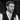# Exploring the Symmetric Difference Interview Question in JavaScript

Nick Scialli January 12, 2020🚀🚀 6 minute read

If you're enjoying this blog, please consider one or both of the following:

# Introduction

The Symmetric Difference interview question is an interesting one as it can be relatively simple to solve if you can think to use the Set object, or, seemingly very challenging or inefficient otherwise.

# The Symmetric Difference Question

First, let’s understand the symmetric difference question. It’s usually posed as some form of the following:

You are asked to create a function that finds the symmetric difference of any number of arrays. The symmetric difference of two arrays is calculated by finding all values that are in one array but not the other array. For example, the symmetric difference of `[1, 2, 3]` and `[2, 3, 4]` is `[1, 4]` since the numbers `1` and `4` are each only in one of the two arrays. Importantly, the output array should only have unique values.

When you have more than two arrays, the symmetric difference is calculate from left to right, comparing the next array with the symmetric difference of the previous two. For example, the symmetric difference of `[1, 2, 3]`, `[2, 3, 4]`, and `[3, 4, 5]` would be calcuated as follows:

• The symmetric difference of `[1, 2, 3]` and `[2, 3, 4]` is `[1, 4]`
• The symmetric difference of `[1, 4]` and `[3, 4, 5]` is `[1, 3, 5]`

Therefore, the answer is `[1, 3, 5]`

## Problem Setup

Based on the problem description, our function description might look something like this:

``````/**
* @param {number[][]} arrs
* @return {number[]}
*/
const symDiff = arrs => {
// Solution here
};``````

Where `arrs` is an array of arrays of numbers, and our output is an array of numbers.

# A Quick Note on Solving Coding Challenges During an Interview

If you’re solving any coding challenge during an interview, it would be prudent to ask some clarifying questions before you start solving the problem. In the symmetric difference case, you might want to ask the following questions (and probably some others I can’t think of):

• Can the input ever be zero arrays? If so, what is the symmetric difference in that case?
• Can the input ever be one array? Again, what would the symmetric difference be in that case?
• Can the input arrays contain anything other than numbers? If so, clarify the behavior in non-number cases.

For the purpose of this blog post, we will assume the input array will always be two or more arrays of numbers.

# An Idiomatic JavaScript Solution

Let’s get right to it: the following snippet shows an idiomatic JavaScript solution that combines concepts like the `Set` object, the `reduce` array method, the ternary operator, and the spread operator.

``````const symDiff = arrs => {
arrs = new Set(arrs);
const diff = arrs.reduce((acc, cur) => {
const prevNums = new Set();
cur.forEach(el => {
if (prevNums.has(el)) return;
});
return acc;
});
return [...diff];
};``````

The real star here is the `Set` object. Let’s dive into how this works.

# How it Works

The best way to see how this works is to step through it line-by-line. I will annotate the previous code with comments, explaining each line.

``````const symDiff = arrs => {
/*
Mutates the first element of the input array
to make it a `Set` object. (Note: it's not
necessarily prudent to mutate your input array,
but we could ask the interviewer if that's
allowed and pivot if it's not).
*/
arrs = new Set(arrs);
/*
Reduce over our input array. The accumulator
(acc) will be start out as our Set above and
then, in each subsequent iterator, will be the
result of the previous symmetric difference!
*/
const diff = arrs.reduce((acc, cur) => {
/*
Create a Set to track if what numbers have
already appeared in the current (cur) array
*/
const prevNums = new Set();
/*
Iterate through each element in the current
array so we can check if it's in the
accumulator array.
*/
cur.forEach(el => {
/*
If this number has already shown up in the
current array, skip it
*/
if (prevNums.has(el)) return;
/*
If the accumulator contains the current
number, then it is in both arrays and cannot
be in the symmetric difference. So, delete it
from the accumulator. On the other hand, if
the current number isn't in the accumulator,
it is in the symmetric difference, so add it.
*/
/*
Take note that this number has been processed
for the current array to make sure we don't
evaluate a duplicate value in the future.
*/
});
/*
We now have our symmetric difference of the
accumulator and the current array! Return the
accumulator for evaluation with the next array
in line (or to return it from the reduce method
if we're done iterating through the arrays)
*/
return acc;
});
/*
Our output has to be an array, so spread the `diff`
set into a new array and return it. Could have
alternatively used `Array.from`.
*/
return [...diff];
};``````

# Conclusion

I like this solution for a couple reasons. It seems to have pretty good time complexity as it requires iterating through the input array-of-arrays exactly once and iterating through each sub-array exactly once. Additionally, it affords you an opportunity to demonstrate knowledge of the `Set` object and to discuss why it’s beneficial to use (namely, that it has hash-table efficiency to look up an element).Nick Scialli is a software engineer at the U.S. Digital Service.

### Subscribe to the mailing list!

If you like what I post here, please sign up to get updates and code insights in your inbox. I won't spam you and you can unsubscribe any time!